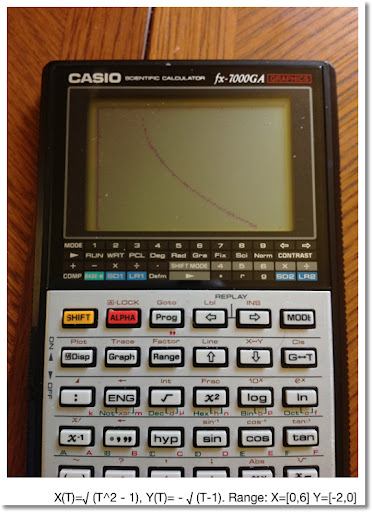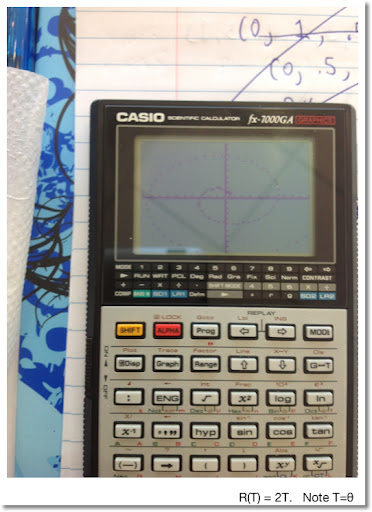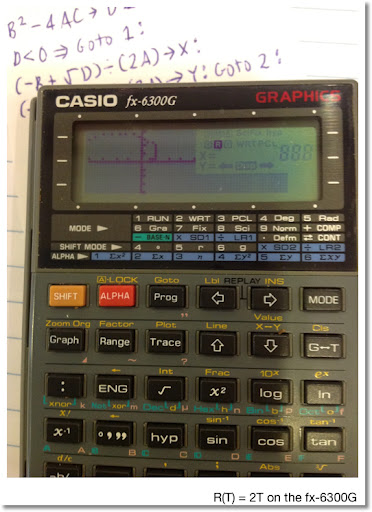## Tuesday, June 19, 2012

### Casio Programming Part II

This blog entry is an extension of the list of programs made with the Casio fx-6300G and the fx-7000GA. Most of the program steps can be transferred verbatim to today's Casio graphing calculators.

Note:
Pol( and Rec( on the fx-6300G and fx-7000GA returns results in variables I and J. I gets r and x, respectively while J gets y and θ, respectively.

The fx-9xxx series (at least the fx-9860 series) returns the conversions from Pol( and Rec( as lists. Adjust accordingly.

However this list of programs will bring more of the complex features offered by today's higher-end calculators.

Here are the links to my two previous posts:

Casio and the Programming Language

Program Library Part 1

The batteries run out on the fx-7000GA fast, or maybe not. It seems to work well after hours of rest.

The programs listed (except for the Quadratic Formula program) on this blog requires a second program, which I designated Program 0. I use program 0 to store my function.

Calculus

Numeric Derivative Approximation

The difference quotient method is used with Δ = 10^-5. Accuracy may vary. Machine accuracy not guaranteed.

Program 0: Enter as a function of X. (example: "2 sin x")

D is the derivative.

Main Program:
"X0"?→Z:
Z + 10^x -5→X: Prog 0: Ans→A:
Z - 10^x -5 → X: Prog 0: Ans→B:
(A - B) ÷ (2 10^x -5) → D

51 steps

Sum of a Function

Σ f(x) from a to b

S is the sum

Program 0: Function of X

Main Program:
"A"?→A:"B"?→B:
A→X: 0→S: Lbl 1:
Prog 0:Ans + S → S:
X + 1 →X:X ≤ B ⇒ Goto 1: S

48 steps

Simpson's Rule - Numeric Integration

I modified the program in the Casio fx-6300G handbook, to try to make it shorter.

N must be even. I is the integral.

Program 0: Function of X

Main Program:
"A"?→ A: "B"?→ B:
"N"?→ N: A→ X: Prog 0: Ans → I:
(B - A) ÷ N → D: N- 2 → O:
Lbl 2:
X + D → X: Prog 0: I + 4 Ans → I:
X + D → X: Prog 0: I + 2 Ans → I:
O - 1 → O: O ≠ 0 ⇒ Goto 2 :
B → X : Prog 0 : (I - Ans) D ÷ 3 → I

113 steps

Graphing: Parametric Equations

Program 0: X(T) to be stored in X, Y(T) to be stored in Y
(Example: 2T sin T→ X: 3T cos T→ Y)

Note: You will have set up the Range in advance which takes intuition, knowledge, and maybe luck to get a "perfect" graph. Once the Range is set up, as I understand it, the graph screen clears.

A = Tmin
B = Tmax
N = number of points

Main Program:
"A"?→A: "B"?→ B:
"N"→ N: (B - A) ÷ N → H: Cls : A - H → T:
Lbl 1: T + H →T:Prog 0: Plot X,Y : T ≠ B ⇒ Goto 1

61 steps

Graphing: Polar Equations

Program 0: R(T) with T being θ
(Example: .5T)

Note: You will have set up the Range in advance which takes intuition, knowledge, and maybe luck to get a "perfect" graph. Once the Range is set up, the graph screen clears.

A = Tmin
B = Tmax
N = number of points

I had better luck with this program on the fx-6300G than on the fx-7000GA.

Main Program:
"A"?→A: "B"?→ B:
"N"→ N: (B - A) ÷ N → H: Cls : A - H → T:
Lbl 1: T + H →T:Prog 0: Rec(Ans,T: Plot I,J : T ≠ B ⇒ Goto 1

67 steps

Note: these are dot plots and are not traceable. I tried using SD2 mode (Scatterplot Mode) but was not able to use comparison commands. Also Line is not the command that it is today.

And now to a favorite program...

AX^2 + BX + C = 0

D = B^2 - 4AC

If D <0, then the roots are complex, X is the real part, Y is the imaginary part, X ± Yi
If D >0, then the roots are X and Y, both real

Program displays D, X, and Y

Preload A, B, and C before running!

B^2 - 4AC → D ◢
D < 0 ⇒ Goto 1:
(-B + √ D) ÷ (2A) → X:
(-B - √ D) ÷ (2A) → Y: Goto 2:
Lbl 1: -B ÷ (2A) → X : √ Abs D ÷ (2A) → Y :
Lbl 2 : X ◢ Y

79 steps

I leave you with some graphs.

Until next time, Eddie

This blog is property of Edward Shore. © 2012### Casio fx-CG50: Sparse Matrix Builder

Casio fx-CG50: Sparse Matrix Builder Introduction The programs can create a sparse matrix, a matrix where most of the entries have zero valu...